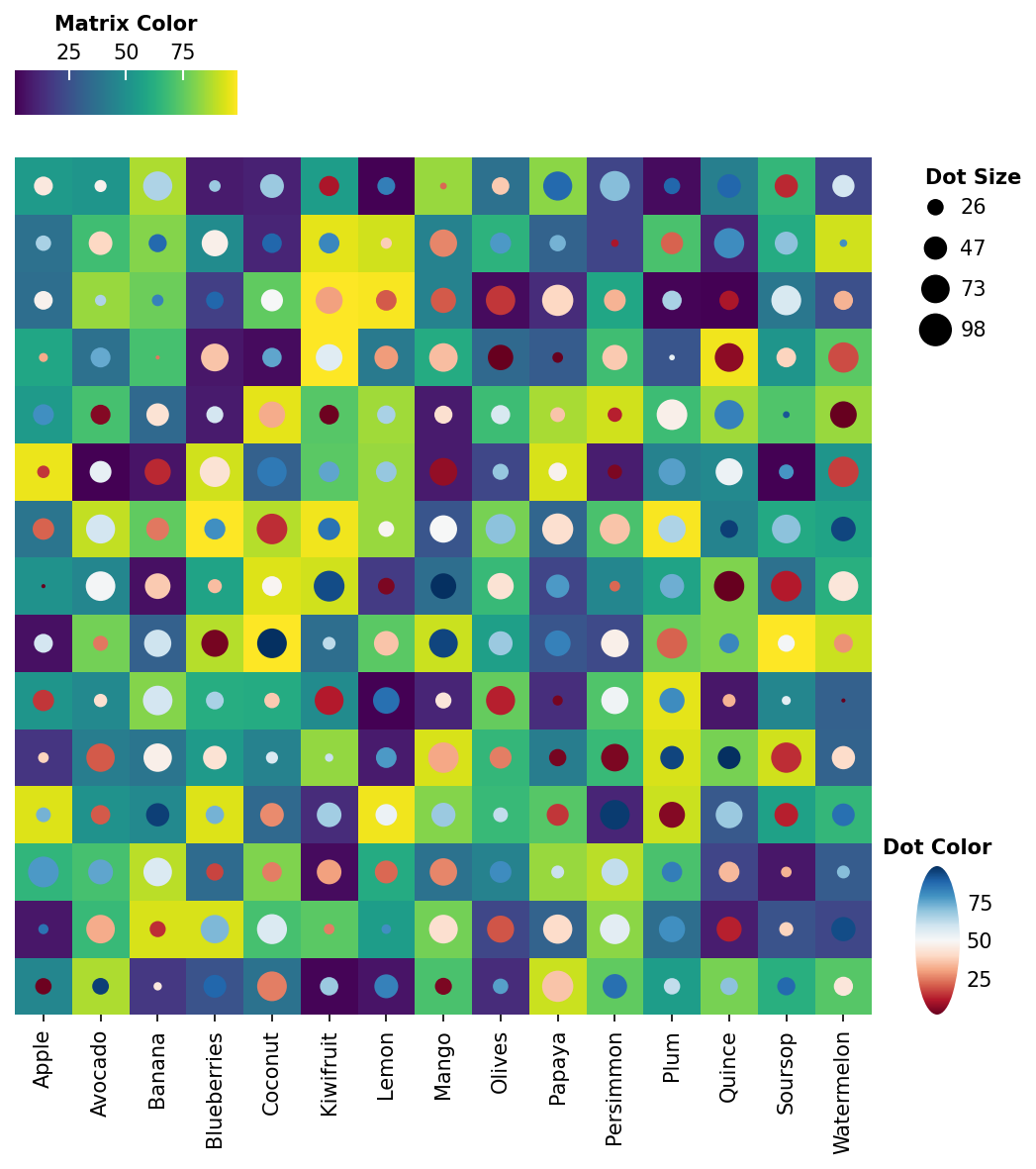# Dot heatmap + Matrix heatmap Example#

Here shows how to draw dot heatmap + matrix heatmap

```import numpy as np
import milkviz as mv
```

## First let’s create some random data#

```np.random.seed(0)
shape = (15, 15)
colors = np.random.randint(1, 100, shape)
sizes = np.random.randint(1, 100, shape)
matrix = np.random.randint(1, 100, shape)
labels = ["Apple", "Avocado", "Banana", "Blueberries", "Coconut", "Kiwifruit", "Lemon",
"Mango", "Olives", "Papaya", "Persimmon", "Plum", "Quince", "Soursop", "Watermelon"]
```

## Create the dot heatmap with the matrix#

```mv.dot_heatmap(sizes, colors, matrix, xticklabels=labels,
dot_size_legend_kw={"title": "Dot Size"},
dot_hue_cbar_kw={"title": "Dot Color"},
matrix_cbar_kw={"title": "Matrix Color"}
)
``````<milkviz._dot_matrix.DotHeatmap object at 0x7f2cb4d0e760>
```

Total running time of the script: ( 0 minutes 1.139 seconds)

Gallery generated by Sphinx-Gallery